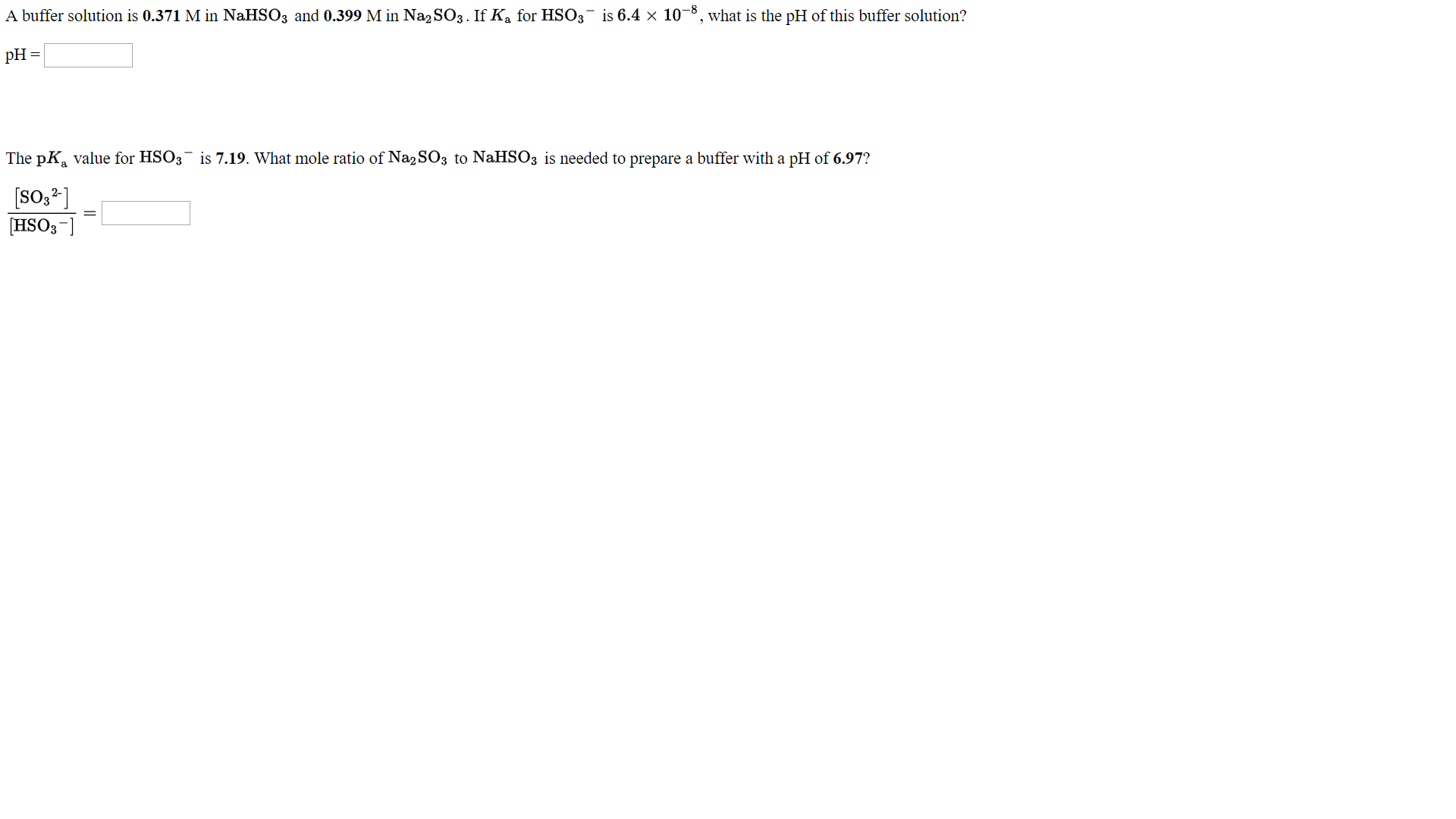A buffer solution is 0.371 M in NaHS03 and 0.399 M in Na2S03. If Ka for HS03-is 6.4 × 10-8, what is the pH of this buffer solution?The pKa value for HSO3 is 7.19. What mole ratio of Na2SO3 to NaHSOs is needed to prepare a buffer with a pH of 6.97?[s032][HS03-]

Questionhelp_outlineImage TranscriptioncloseA buffer solution is 0.371 M in NaHS03 and 0.399 M in Na2S03. If Ka for HS03-is 6.4 × 10-8, what is the pH of this buffer solution? The pKa value for HSO3 is 7.19. What mole ratio of Na2SO3 to NaHSOs is needed to prepare a buffer with a pH of 6.97? [s032] [HS03-] fullscreen
Step 1

The equilibrium reaction can be represented as,

Step 2

Calculate the pH of the given buffer solution using Henderson-Hasselbalch equation.

The Ka of HSO3- is 6.4*10-8

Step 3

Use Henderson-Hasselbalch equation to find the mole ratio o...

Want to see the full answer?

See Solution

Want to see this answer and more?

Our solutions are written by experts, many with advanced degrees, and available 24/7

See Solution
Tagged in

Physical Chemistry